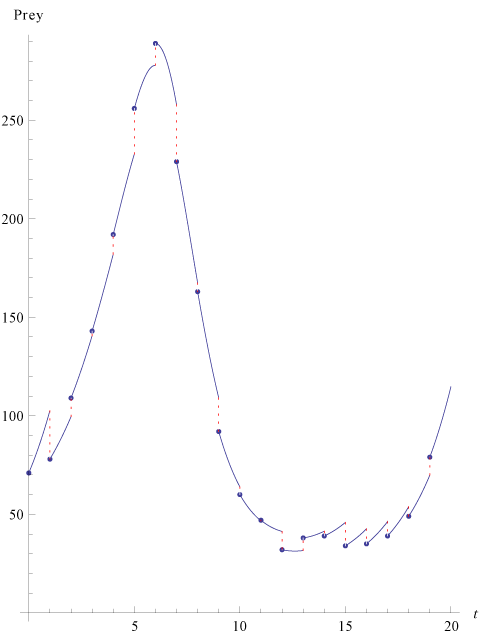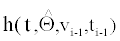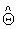Figure 3: Representation of Lotka-Volterra system’s dynamics with MSS method. The blue points are the data points. Blue curves are the ODE dynamics for each time interval[ti-1,ti] namelywith the estimated parameter. Red dotted lines show the residuals. 40 simulated observations from partially observed Lotka-Volterra model (prey) with Θ=(0.5,0.0025,0.3), T=40 and v0=(71,79) for the estimation ofusing (2) with K={0}. Dynamics shown until T=20.